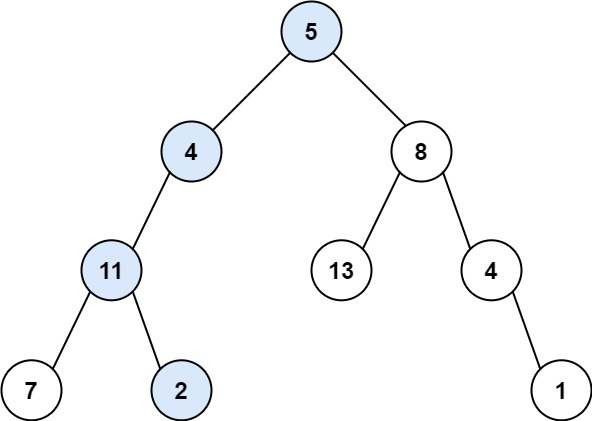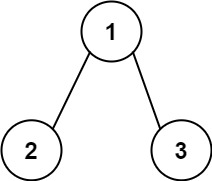# LeetCode: 112. Path Sum

## 题目¶

Given the root of a binary tree and an integer targetSum, return true if the tree has a root-to-leaf path such that adding up all the values along the path equals targetSum.

A leaf is a node with no children.

Example 1:```Input: root = [5,4,8,11,null,13,4,7,2,null,null,null,1], targetSum = 22
Output: true
```

Example 2:```Input: root = [1,2,3], targetSum = 5
Output: false
```

Example 3:

```Input: root = [1,2], targetSum = 0
Output: false
```

Constraints:

• The number of nodes in the tree is in the range [0, 5000].
• -1000 <= Node.val <= 1000
• -1000 <= targetSum <= 1000

## 解法¶

```# Definition for a binary tree node.
# class TreeNode(object):
#     def __init__(self, val=0, left=None, right=None):
#         self.val = val
#         self.left = left
#         self.right = right
class Solution(object):
def hasPathSum(self, root, targetSum):
if root is None:
return False

if root.left is None and root.right is None and root.val == targetSum:
return True

new_sum = targetSum - root.val

return self.hasPathSum(root.left, new_sum) or self.hasPathSum(root.right, new_sum)
```